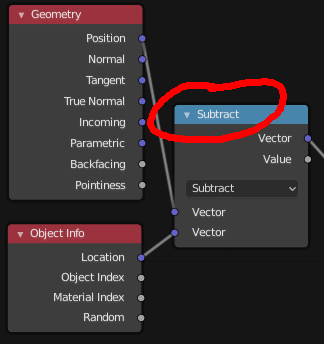# Math nodes shouldn't change their titles to their operation type name

Right now, the Math and Vector Math nodes adapt their title to their current operation name. For example, the Subtract operation:Even though this is a Subtract operation selected from the dropdown of a Vector Math node, this node title implies that the actual node is a Subtract node which does not exist. For consistency, a node should really say its own name, not change with the values in the node. This is also redundant because it says the operation selection twice, right above and below each other. This also makes it harder to tell from a glance whether, for example, “Subtract” refers to vector math or scalar math because it doesn’t say the node type.

I would propose removing the dynamic title and keeping the actual node name consistent so it isn’t so funky.

9 Likes

That is the real problem. Theme is giving same color to all nodes of Convertor category.
Math nodes and Vector nodes are using same color for label.
There is one difference that user can take attention to. It is color of socket.
Blue sockets for Vectors. Grey sockets for values.
So, if all sockets are grey : it is a math node.

We could imagine to add a prefix for vector nodes instead of removing a useful feature.
But I would really prefer that an exception would be done to colorization by category for this type of nodes.

It is only the case if node is open. But to gain space and clarity those kind of nodes are often closed by users. At that moment, it is really useful to perceive operation into title of node.
That is an automatic label. But if user wants to precise a label that is not dynamic, he can give a static name into node properties. When a label is precised by user, it does not change if operation is changed.

`self.label = "Math: Subtract"`